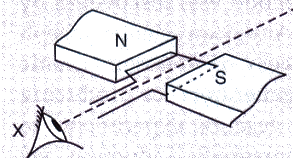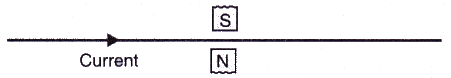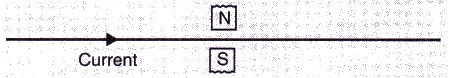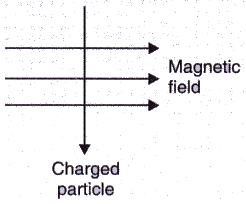Courses

# Solutions of Magnetic Effects of Electric Current (Page No- 93) - Physics By Lakhmir Singh, Class 10 Class 10 Notes | EduRev

## Class 10 : Solutions of Magnetic Effects of Electric Current (Page No- 93) - Physics By Lakhmir Singh, Class 10 Class 10 Notes | EduRev

The document Solutions of Magnetic Effects of Electric Current (Page No- 93) - Physics By Lakhmir Singh, Class 10 Class 10 Notes | EduRev is a part of Class 10 category.
All you need of Class 10 at this link: Class 10

Lakhmir Singh Physics Class 10 Solutions Page No:93

Question 31: In the simple electric motor of figure given below, the coil rotates anticlockwise as seen by the eye from the position X when current flows in the coil. Is the current flowing clockwise or anticlockwise around the coil when viewed from above ?Solution : Clockwise direction (according to Fleming’s left hand rule).

Question 32: Which way does the wire in the diagram below tend to move ?Solution : According to Fleming’s left hand rule, the wire moves in the upward direction (out of the page).

Question 33: If the current in a wire is flowing in the vertically downward direction and a magnetic field is applied from west to east, what is the direction of force on the wire ?

Solution : Force will be due South (according to Fleming’s left hand rule).

Question 34: Which way does the wire in the diagram below tend to move ?Solution : According to Fleming’s left hand rule, the wire moves in the downward direction (into the page).

Question 35: What is the force on a current-carrying wire that is parallel to a magnetic field ? Give reason for your answer.

Solution : Force on a current-carrying wire that is parallel to magnetic field will be zero.
This is because the magnitude of force depends on the sin of the angle between the direction of current and the direction of magnetic field, so if the current carrying wire is held parallel to the magnetic field, the force will be zero.

Question 36: A charged particle enters at right angles into a uniform magnetic field as shown :
What should be the nature of charge on the particle if it begins to move in a direction pointing vertically out of the page due to its interaction with the magnetic field ?Solution : Positive charge.

Offer running on EduRev: Apply code STAYHOME200 to get INR 200 off on our premium plan EduRev Infinity!

,

,

,

,

,

,

,

,

,

,

,

,

,

,

,

,

,

,

,

,

,

,

,

,

;